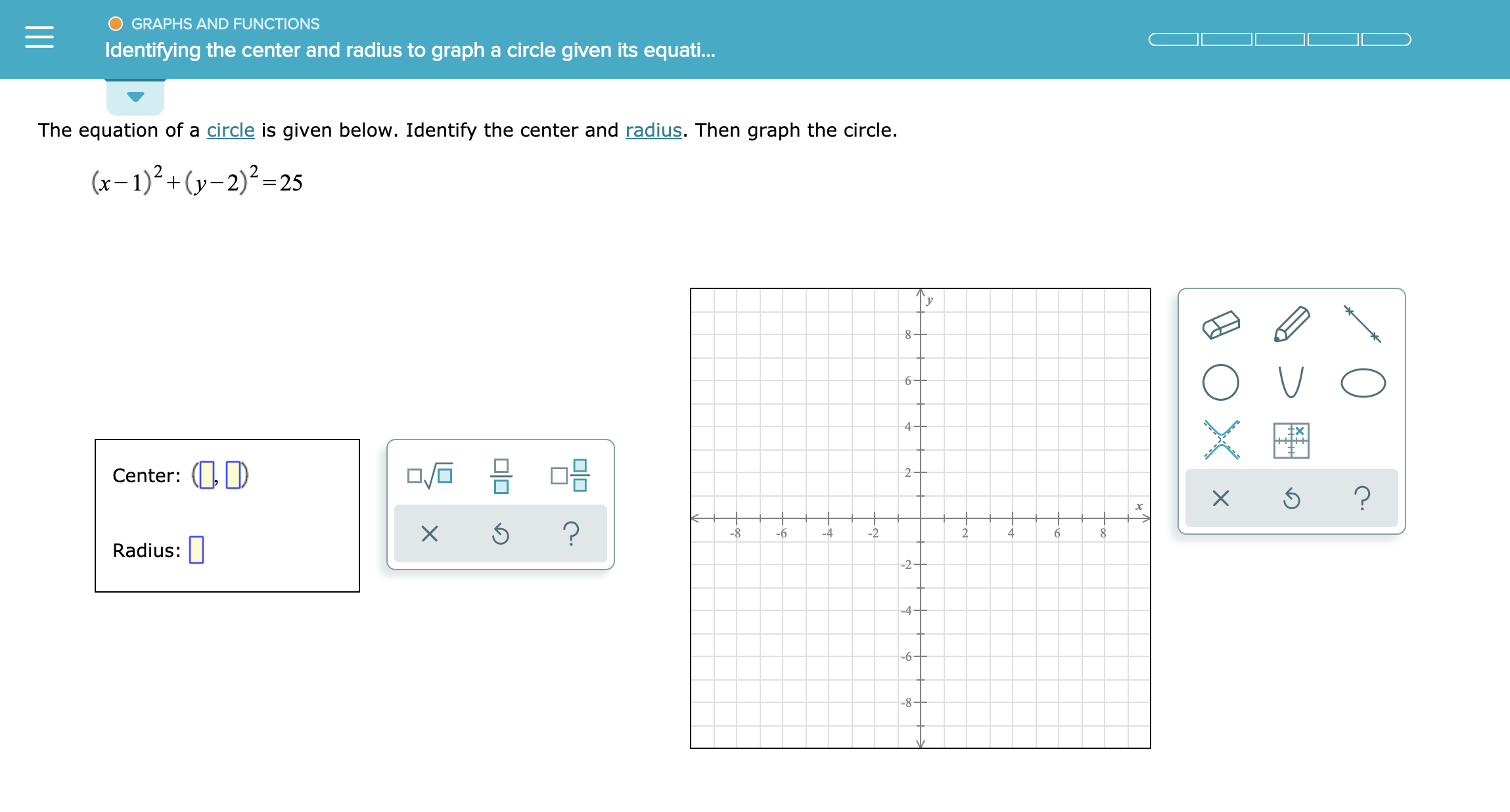# GRAPHS AND FUNCTIONS Identifying the center and radius to graph a circle given its equati... The equation of a circle is given below. Identify the center and radius. Then graph the circle. (-)2+(y2)225 _ 8 6 4 Center: ? ? -8 -6 -2 -4 4 Radius: -2 -4 6 -8- X X II

Question

See attachedhelp_outlineImage TranscriptioncloseGRAPHS AND FUNCTIONS Identifying the center and radius to graph a circle given its equati... The equation of a circle is given below. Identify the center and radius. Then graph the circle. (-)2+(y2)225 _ 8 6 4 Center: ? ? -8 -6 -2 -4 4 Radius: -2 -4 6 -8- X X II fullscreen

### Want to see this answer and more?

Experts are waiting 24/7 to provide step-by-step solutions in as fast as 30 minutes!*

*Response times vary by subject and question complexity. Median response time is 34 minutes and may be longer for new subjects.
Tagged in
MathCalculus

### Other